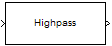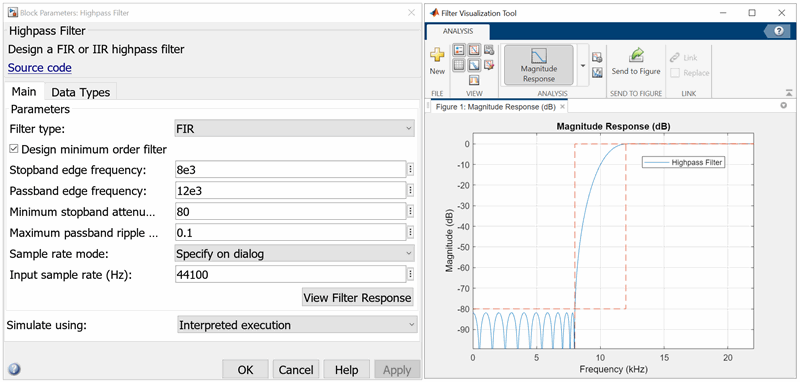# Highpass Filter

Design FIR or IIR highpass filter

•Libraries:
DSP System Toolbox / Filtering / Filter Designs

## Description

The Highpass Filter block independently filters each channel of the input signal over time using the given design specifications. You can control whether the block implements an IIR or FIR highpass filter using the Filter type parameter. You can specify the passband and stopband edge frequencies in Hz or in normalized frequency units (since R2023a).

This block supports ARM® Cortex® code generation. Under certain conditions, this block also supports SIMD code generation. For details, see Code Generation.

## Ports

### Input

expand all

Input signal, specified as a real- or complex-valued column vector or matrix. If the input signal is a matrix, each column of the matrix is treated as an independent channel. The number of rows in the input signal denotes the channel length.

Data Types: `single` | `double` | `fixed point`
Complex Number Support: Yes

### Output

expand all

Filtered signal, returned as a vector or matrix. The output has the same size and complexity characteristics as the input. If the output has a fixed-point data type, it is always signed.

Data Types: `single` | `double` | `fixed point`
Complex Number Support: Yes

## Parameters

expand all

### Main

Specify whether the block implements an FIR highpass filter or an IIR highpass filter.

When you select this check box, the block designs a filter with minimum order. When you clear this check box, you can specify the Filter order as a positive integer.

Specify the order of the highpass filter as a positive integer.

#### Dependencies

To enable this parameter, clear the Design minimum order filter check box.

Specify the stopband edge frequency of the highpass filter as a real positive scalar in Hz or in normalized frequency units (since R2023a).

If you set the Sample rate mode parameter to:

• `Specify on dialog` or `Inherit from input port` –– The value of the stopband edge frequency is in Hz and must be less than the passband edge frequency.

• ```Use normalized frequency (0 to 1)``` –– The value of the stopband edge frequency is in normalized frequency units. The value must be a positive scalar less than the passband edge frequency.

(since R2023a)

#### Dependencies

To enable this parameter, select the Design minimum order filter check box.

Specify the passband edge frequency of the highpass filter as a real positive scalar in Hz or in normalized frequency units (since R2023a).

If you set the Sample rate mode parameter to:

• `Specify on dialog` or `Inherit from input port` –– The value of the passband edge frequency is in Hz and must be less than half the value of the input sample rate.

• ```Use normalized frequency (0 to 1)``` –– The value of the passband edge frequency is in normalized frequency units. The value must be a positive scalar less than `1.0`.

(since R2023a)

Specify the minimum attenuation in the stopband as a real positive scalar in dB.

Specify the maximum ripple of the filter response in the passband as a real positive scalar in dB.

Since R2023a

Specify the input sample rate using one of these options:

• `Specify on dialog` –– Specify the input sample rate in the block dialog box using the Input sample rate (Hz) parameter.

• `Inherit from input port` –– The block inherits the sample rate from the input signal.

• ```Use normalized frequency (0 to 1)``` –– Specify the passband edge and the stopband edge frequencies in normalized frequency units (0 to 1).

Specify the input sample rate as a scalar in Hz.

#### Dependencies

To enable this parameter, set the Sample rate mode parameter to `Specify on dialog`. (since R2023a)

Type of simulation to run:

• `Interpreted execution` (default)

Simulate model using the MATLAB® interpreter. This option shortens startup time but has slower simulation speed than ```Code generation```.

• `Code generation`

Simulate model using generated C code. The first time you run a simulation, Simulink® generates C code for the block. The C code is reused for subsequent simulations as long as the model does not change. This option requires additional startup time but provides faster simulation speed than `Interpreted execution`.

Opens the Filter Visualization Tool (`fvtool`) and displays the magnitude/phase response of the highpass filter. The response is based on the block dialog box parameters. Changes made to these parameters update FVTool.To update the magnitude response while FVTool is running, modify the dialog box parameters and click .

### Data Types

Rounding method for the output fixed-point operations.

Fixed-point data type of the coefficients, specified as one of the following:

• `fixdt(1,16)` — Signed fixed-point data type of word length `16`, with binary point scaling. The block determines the fraction length automatically from the coefficient values in such a way that the coefficients occupy maximum representable range without overflowing.

• `fixdt(1,16,0)` — Signed fixed-point data type of word length `16` and fraction length `0`. You can change the fraction length to any other integer value.

• `<data type expression>` — Specify the data type using an expression that evaluates to a data type object, for example, numeric type (`fixdt`(```[ ]```,`16`, `15`)). Specify the sign mode of this data type as `[ ]` or `true`.

• `Refresh Data Type` — Refresh to the default data type.

Click the buttonto display the data type assistant, which helps you set the data type. For more information, see Specify Data Types Using Data Type Assistant (Simulink).

## Block Characteristics

 Data Types `double` | `fixed point` | `integer` | `single` Direct Feedthrough `no` Multidimensional Signals `no` Variable-Size Signals `yes` Zero-Crossing Detection `no`

expand all

## Version History

Introduced in R2015b

expand all